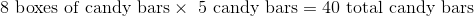# ISEE Lower Level Math : How to multiply

## Example Questions

← Previous 1 3 4 5 6 7 8 9

### Example Question #1 : Operations

Susie needs to buy new supplies for school. Pencils are sold for 23 cents a piece. Notebooks are sold in pairs, at $3.04 a pair. Folders are sold in packages of 4, and each package costs$2.12.

If Susie buys a 7 pencils, 8 notebooks, and a dozen folders with $25, how much change should she receive? Possible Answers: Susie does not have enough money to purchase all of these school supplies.$2.75

$4.78$4.87

$8.83 Correct answer:$4.87

Explanation:

Pencils are sold for $0.23 each. If Susie buys 7 pencils, she is paying 7 x$0.23 =

$1.61 for pencils. Notebooks are sold in pairs (or in packages of two), for$3.04 a pair.

If Susie buys 8 notebooks, that means that she bought

8 ÷ 2 =

4 pairs of notebooks.

So, 4 pairs of notebooks, at $3.04 a pair costs 4 x$3.04 =

$12.16. Susie also buys a dozen, or 12, folders. Folders come in packages of 4. This means that Susie buys 12 ÷ 4 = 3 packages of folders. Because each package costs$2.12, Susie pays

3 x $2.12 =$6.36 for a dozen folders.

If we add up the total cost of the pencils, notebooks, and folders we get

$1.61 +$12.16 + $6.36 =$20.13.

Because Susie pays $25, she will be left with$25 – $20.13 =$4.87 in change.

### Example Question #2 : Operations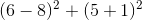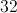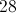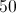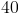Explanation: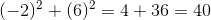### Example Question #3 : How To Multiply

Solve using the order of operations: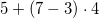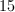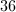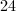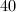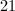Explanation:

The order of operations states that we must begin solving the mathematical expression by evaluating numbers in parentheses. In this expression,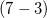is in parentheses. When we solve this, we get. Our expression can now be rewritten as: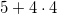The order of operations states that the next step would be to evaluate any term with an exponent. Since there are no exponents in this expression, we move on to multiplication. In this expression we are asked to multiply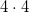, which is. Our expression can now be written as: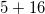The order of operations would have us divide next, but seeing as there is no division in this expression, we move on to addition.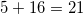.

### Example Question #1 : Operations

Read the following problem but do not solve it.

Acording to Mr. Smith's will, when he dies, each of his six children is to be left a parcel of land 510 acres in area. If these six parcels make up all of the farm, then how large is Mr. Smith's farm now?

Which of the following expressions must be evaluated in order to answer this question?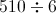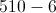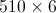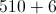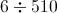Explanation:

There are six parcels of equal size; the total size is therefore the product of the common size (510 acres) and the number of parcels (6). This is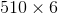### Example Question #5 : How To Multiply

Evaluate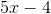for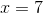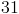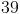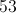Explanation:

Substitute 7 for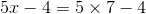By order of operations, multiply, then subtract.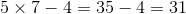### Example Question #3 : Operations

Evaluate: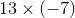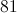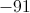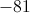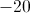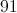Explanation:

To multiply two numbers of unlike sign, multiply their absolute values, then affix a negative symbol in front: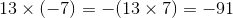### Example Question #4 : Operations

Evaluate: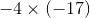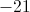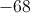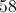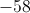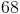Explanation:

To multply two negative numbers, just multiply their absolute values; the product will be positive: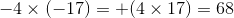### Example Question #8 : How To Multiply

Evaluate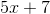for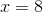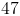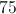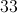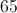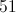Explanation:

Substitute 8 for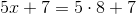By order of operations, multiply, then add.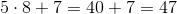### Example Question #5 : Operations

Find the product.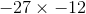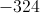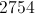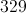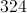Explanation:

When multiplying two negative numbers, the product will be positive. The correct answer is 324.

### Example Question #6 : Operations

Which story best fits the equation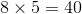?

I have 40 candy bars. If I eat 8, how many are left?

I have 8 candy bars, and my friend has 5. How many do we have together?

I want to share 40 candy bars with 10 friends. How many do they each get?

I have 8 boxes of candy bars, and each box has 5 candy bars. How many bars do I have in total?